### Home > PC > Chapter 4 > Lesson 4.3.2 > Problem4-138

4-138.
1. Find all values of θ where 0 ≤ θ < 2π, such that the following equations are true. Use exact values for θ. Homework Help ✎

1. cos θ = 1

2. tan θ = −1

3. csc θ = 2

4. sec θ =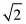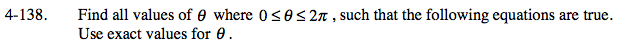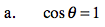Cosine is the x-value. Where on the unit circle is x = 1?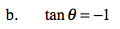What θ gives a tangent of 1 or −1?
What quadrants would the tangent be −1?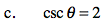Change the csc θ to 1/sin θ.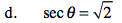$\frac{1}{\cos\theta}=\frac{\sqrt2}{1}$